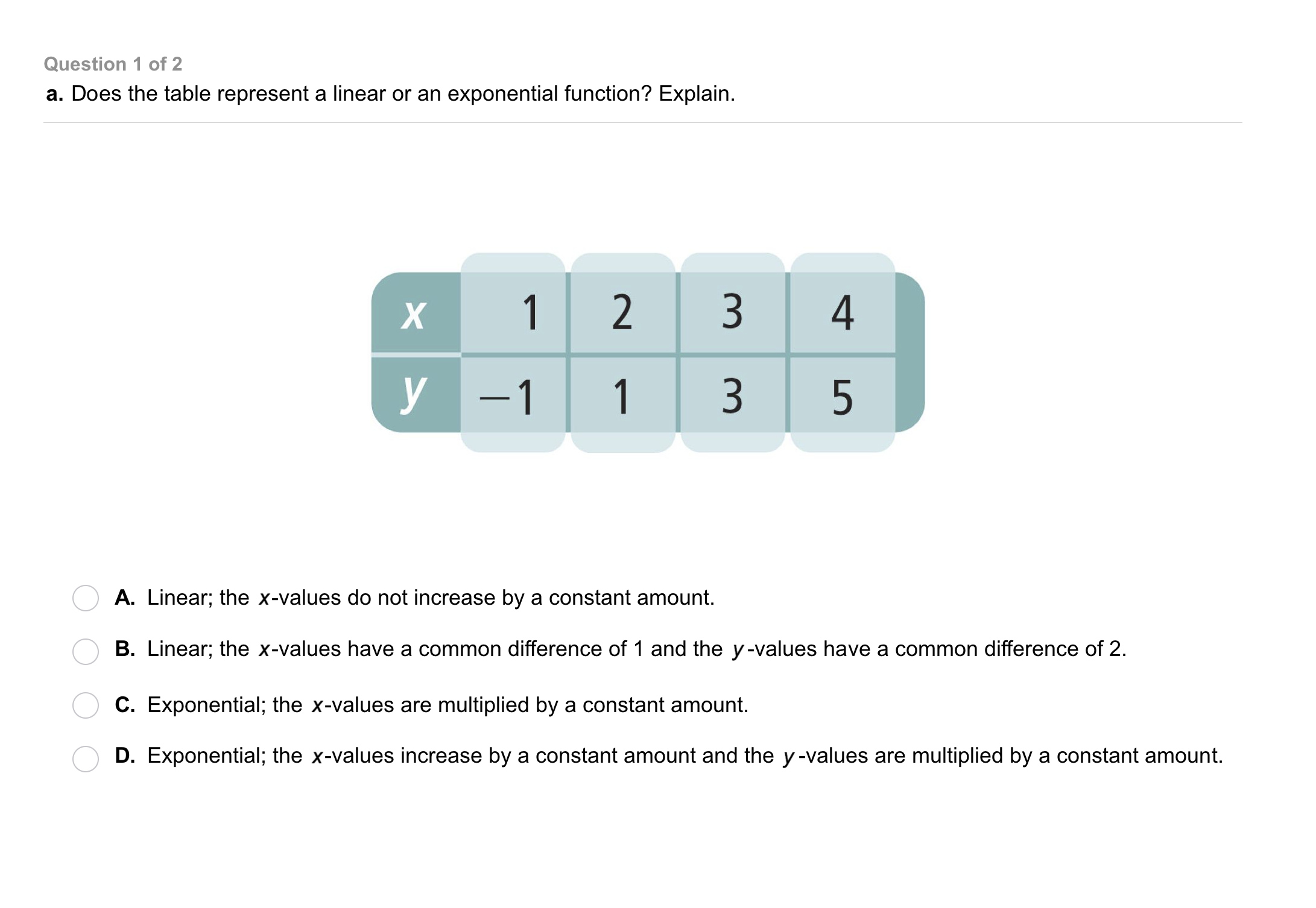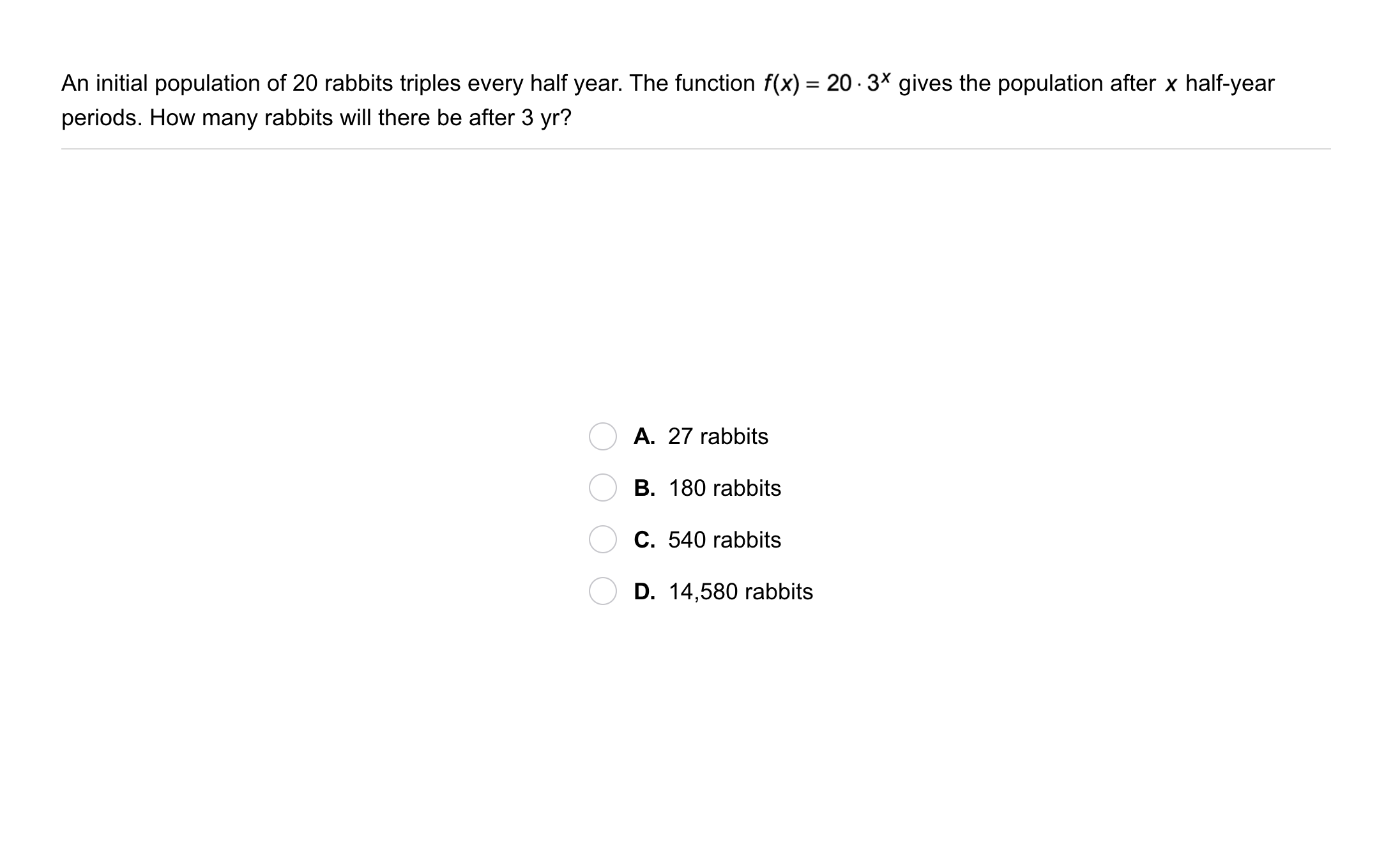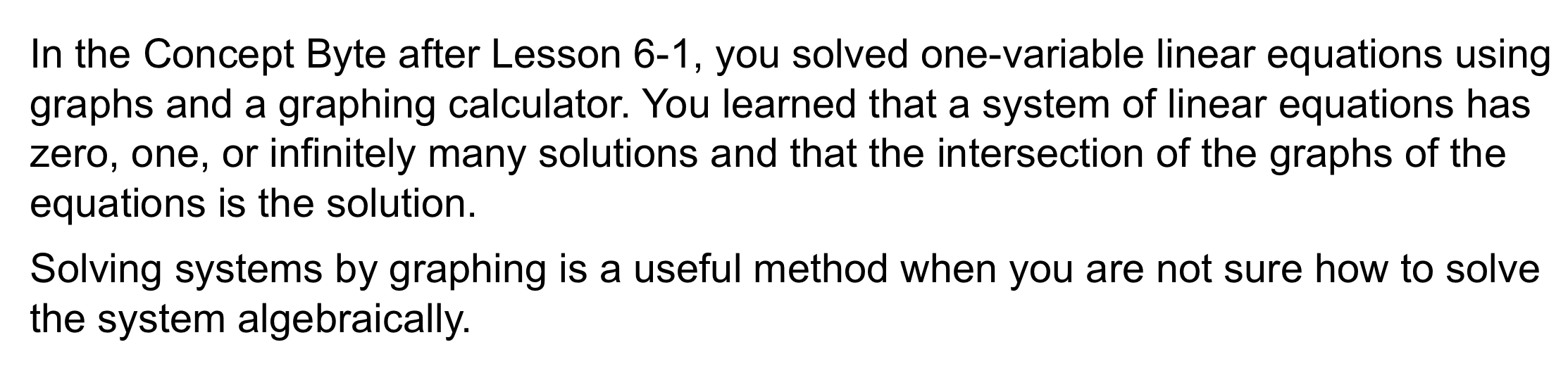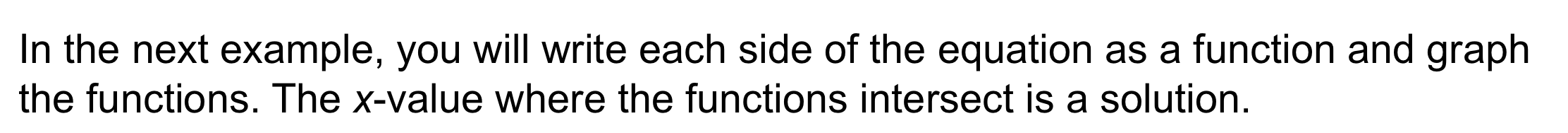Algebra 1 7-6 Complete Lesson: Exponential Functions
starstarstarstarstarstarstarstarstarstar
5 (1 rating)
by Matthew Richardson
| 31 Questions
Note from the author:
A complete formative lesson with embedded slideshow, mini lecture screencasts, checks for understanding, practice items, mixed review, and reflection. I create these assignments to supplement each lesson of Pearson's Common Core Edition Algebra 1, Algebra 2, and Geometry courses. See also mathquest.net and twitter.com/mathquestEDU.The outlined content above was added from outside of Formative.1
2
1
5 pts
Solve It! Your soccer team wants to practice a drill for a certain amount of time each day. Which plan will give your team more total practice time over 4 days?
Plan 1
Plan 2
2
5 pts
Solve It! Which plan will give your team more total practice over 8 days?
Plan 1
Plan 23
3
10 pts
Problem 1 Got It?
A
B
C
D4
4
10 pts
Problem 1 Got It?
A
B
C
D5
5
10 pts
Problem 2 Got It?
A
B
C
D6
10 pts
Problem 3 Got It? Graph the function on the coordinate plane.
Be sure to include relevant graph detail: label axes, indicate units and scale on both axes, and use arrows to represent end behavior, as appropriate.
7
10 pts
Problem 3 Got It? Graph the function on the coordinate plane.
Be sure to include relevant graph detail: label axes, indicate units and scale on both axes, and use arrows to represent end behavior, as appropriate.
8
10 pts
Problem 4 Got It? You can also zoom out to view a larger area on the map in Problem 4. The function models the percent of the original area the map shows after zooming out x times.
Graph the function. Zoom the graphing utility to establish an appropriate viewing window.
9
10 pts
Problem 4 Got It? Reasoning: What is the percent increase in the area each time you zoom out in the previous item? Enter only a number.10
10
10 pts
Problem 5 Got It?
A
B
C
D11
11
10 pts
Problem 5 Got It?
A
B
C
D12
12
10 pts
Problem 5 Got It?
A
B
C
D13
10 pts
Identify the domain and range of the relation {(-2, 3), (-1, 4), (0, 5), (1, 6)}.
Represent the relation with a mapping diagram by adding the domain, range, and arrows to the template provided.14
14
10 pts
Is the relation in the graph a function?
Use the vertical line test.
No, it is not a function. The graph fails the vertical line test.
Yes, it is a function. The graph passes the vertical line test.
15
10 pts
What is f(2) for the function?

16
10 pts
The domain of f(x) is {-4, -2, 0, 2, 4}
Given the domain above, what is the range?
{-2, -1, 0, -1, -2}
{-4, -2, 0, 2, 4}
{-2, -1, 0, 1, 2}18
10 pts
Reasoning: Is the function an exponential function? Justify your answer.19
19
3 pts

Error Analysis: A student evaluated the function f(x) for x = -1 as shown. Describe and correct the student's mistake.20
10 pts
Review Lesson 7-4: Simplify the expression.

21
10 pts
Review Lesson 7-4: Simplify the expression.

22
10 pts
Review Lesson 7-4: Simplify the expression.

23
10 pts
Review Lesson 5-6: Write an equation for the line that is parallel to the given line and passes through the given point.
y = 5x + 1
y = x + 5
y = 5x
y = x
24
10 pts
Review Lesson 5-6: Write an equation for the line that is parallel to the given line and passes through the given point.
y = 3x + 1
y = 3x
y = x
y = 2x + 3
25
10 pts
Review Lesson 2-10: Identify each scenario as representing percent increase or percent decrease.
• original price: \$25; sale price: \$22
• height last week: 15 cm; height this week: 18 cm
• price this year: \$999; price last year: \$1450
• birth weight: 220 lb; weight at 1 month: 300 lb
• Percent increase
• Percent decrease
26
10 pts
Review Lesson 2-10: Identify each percent change. Round to the nearest percent.
• original price: \$25; sale price: \$22
• height last week: 15 cm; height this week: 18 cm
• price this year: \$999; price last year: \$1450
• birth weight: 220 lb; weight at 1 month: 300 lb
• 31%
• 20%
• 36%
• 12%27
10 pts
Vocabulary Review: Identify each relation based on whether or not it is a function.
• {(1, 3), (1, 5), (2, 7), (3, 9), (4, 11)}
• {(0, 0), (-1, -1), (-2, -2), (-3, -3)}
• {(5, 5), (5, 4), (5, 3), (5, 4)}
• {(2, 5), (3, 5), (4, 5), (5, 5)}
• Function
• Not a function28
10 pts
Use Your Vocabulary: Complete the sentence with the word exponential or exponent. Respond with only the word.

In the expression 5³, 3 is the __?__.
29
10 pts
Use Your Vocabulary: Complete the sentence with the word exponential or exponent. Respond with only the word.
The expression above is an __?__ expression.
30
100 pts
Notes: Take a clear picture or screenshot of your Cornell notes for this lesson. Upload it to the canvas. Zoom and pan as needed.

For a refresher on the Cornell note-taking system, click here.
31
10 pts
Reflection: Math Success
Add to my formatives list

Formative uses cookies to allow us to better understand how the site is used. By continuing to use this site, you consent to the Terms of Service and Privacy Policy.